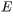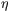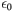# Problem: Learning Goal: To understand the behavior of the electric field at the surface of a conductor, and its relationship to surface charge on the conductor.A conductor is placed in an external electrostatic field. The external field is uniform before the conductor is placed within it. The conductor is completely isolated from any source of current or charge.PART A) Which of the following describes the electric field inside this conductor?A. It is in the same direction as the original external field.B. It is in the opposite direction from that of the original external field.C. It has a direction determined entirely by the charge on its surface.D. It is always zero.PART B) The charge density inside the conductor is:A. 0B. non-zero; but uniformC. non-zero; non-uniformD. infinitePART C) Assume that at some point just outside the surface of the conductor, the electric field has magnitude and is directed toward the surface of the conductor. What is the charge density  on the surface of the conductor at that point?Express your answer in terms of and . =

###### FREE Expert Solution

Electric flux:

$\overline{){\mathbf{\varphi }}{\mathbf{=}}\frac{\mathbf{q}}{{\mathbf{\epsilon }}_{\mathbf{0}}}}$

$\overline{){\mathbf{\varphi }}{\mathbf{=}}{\mathbf{E}}{\mathbf{A}}{\mathbf{c}}{\mathbf{o}}{\mathbf{s}}{\mathbf{\theta }}}$

Charge density:

$\overline{){\mathbf{\rho }}{\mathbf{=}}\frac{\mathbf{q}}{\mathbf{V}}}$

Part (A)

If the conductor is in the external electric field, the charges in the conductor will be rearranged.

It is these rearranged charges that produce an electric field, which has the same magnitude but in the opposite direction to the external electric field.

89% (12 ratings)###### Problem Details

Learning Goal: To understand the behavior of the electric field at the surface of a conductor, and its relationship to surface charge on the conductor.

A conductor is placed in an external electrostatic field. The external field is uniform before the conductor is placed within it. The conductor is completely isolated from any source of current or charge.

PART A) Which of the following describes the electric field inside this conductor?

A. It is in the same direction as the original external field.

B. It is in the opposite direction from that of the original external field.

C. It has a direction determined entirely by the charge on its surface.

D. It is always zero.

PART B) The charge density inside the conductor is:

A. 0

B. non-zero; but uniform

C. non-zero; non-uniform

D. infinite

PART C) Assume that at some point just outside the surface of the conductor, the electric field has magnitudeand is directed toward the surface of the conductor. What is the charge densityon the surface of the conductor at that point?

Express your answer in terms ofand.=